# MCQs on Electric Circuits

##### Page 55 of 63. Go to page 1 2 3 4 5 6 7 8 9 10 11 12 13 14 15 16 17 18 19 20 21 22 23 24 25 26 27 28 29 30 31 32 33 34 35 36 37 38 39 40 41 42 43 44 45 46 47 48 49 50 51 52 53 54 55 56 57 58 59 60 61 62 63
01․ The maximum value of a current wave is 2A. If the form factor of a current wave is 1.5 and the peak factor is 2.7, find the rms value in Amperes.
0.494 A.
0.741 A.
1.57 A.
1.414 A.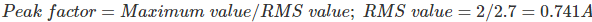02․ The maximum value of a current wave is 2A. If the form factor of a current wave is 1.5 and the peak factor is 2.7, find the average value in Amperes.
1.414 A.
0.494 A.
0.741 A.
1.57 A.03․ The average value of half wave rectified sinusoidal current waveform, whose maximum value is 1A is
0.5 A.
1 A.
0.318 A.
0.637 A.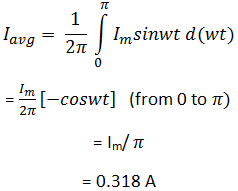04․ The average value of half wave rectified sinusoidal current waveform, whose maximum value is 1A is
0.5A.
1A.
0.318A.
0.637A.

The RMS value of any signal u(t) is given by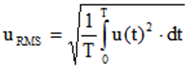Where, T is the time period of the signal Now,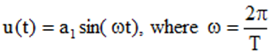Where, a1 is the peak value of the sinusoidal signal and ω is the angular frequency. So, for half wave rectified sinusoidal current waveform, the value is zero from π to 2π.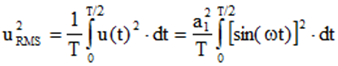Therefore,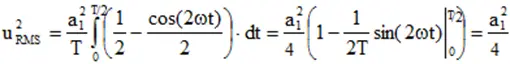So, the RMS value uRMS = a1/2 = 0.5 times the peak value.

05․ A 230V, 50Hz voltage is applied to a coil having L = 5H and R = 2 Ohm in series with capacitance C. When the voltage across the coil is 400V, find the current in the circuit?
0.1465 A.
0.1578 A.
0.2547 A.
0.4583 A.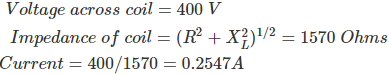06․ A 230V, 50Hz voltage is applied to a coil having L = 5H and R = 2 Ohm in series with capacitance C. When the voltage across the coil is 400V, find the value of C?
10.54 µF.
8.51 µF.
1.8 µF.
4.78 µF.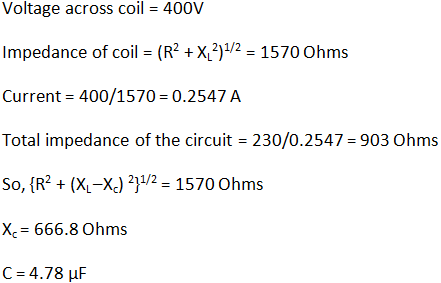07․ A series RLC circuit is connected across a 240V, 50 Hz supply. When the voltage across capacitor is 250V, a maximum current of 0.5A flows in the circuit. Find the resistance.
480 Ohms.
500 Ohms.
100 Ohms.
1000 Ohms.

In series RLC circuit, maximum current flows when the inductance and capacitance cancels out the effect of each other and the circuit is completely resistive. So, R = 240/0.5 = 480 Ohms.

08․ A series RLC circuit is connected across a 240V, 50 Hz supply. When the voltage across capacitor is 250V, a maximum current of 0.5A flows in the circuit. Find the inductance.
2.25 µF.
6.37 µF.
1.58 µF.
0.47 µF.

In series RLC circuit, maximum current flows when the inductance and capacitance cancels out the effect of each other and the circuit is completely resistive. Voltage across capacitance is 250V. Capacitive reactance (Xc) = 250/0.5 = 500 Ohms.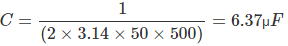09․ A series RLC circuit is connected across a 240V, 50 Hz supply. When the voltage across capacitor is 250V, a maximum current of 0.5A flows in the circuit. Find the inductance.
2.55 H.
1.95 H.
1.59 H.
0.45 H.

In series RLC circuit, maximum current flows when the inductance and capacitance cancels out the effect of each other and the circuit is completely resistive. So the capacitive reactance and inductive reactance are same.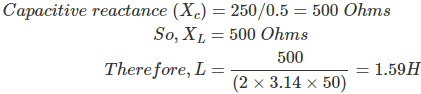10․ A dependent source can be
a current source.
a voltage source.
neither a voltage nor a current source.
both a voltage or a current source.

A dependent source can be a voltage source or a current source, the value of which is a function of voltage or current in some other part of the circuit.

<<<5354555657>>>